# 5 Introduction of sources and PCB layout structures to the cavity model

Efficient power integrity analysis with cavity models for rectangular power planes obtained from (4.13) and (4.14) have been presented by  and . While power-ground planes are excited by currents, which are galvanically supplied to the planes, an enclosure is excited by common mode coupling from a PCB trace to the cavity between the ground plane and the cover. Since the models (4.13) and (4.14) can only be excited by currents supplied to the planes, the common mode coupling of a trace also has to be introduced into these models by current sources, connected to the upper and lower plane.
According to conditions (4.4) and (4.5) only the TMzm (magnetic field transversal in the z direction) mode m=0 is considered in the cavity model and the electric field has thus only a z-component. This is also sufficient in case of a trace within the cavity, because higher order parallel plate modes, excited by the horizontal trace current, decay rapidly and cannot reach the surrounding edges. Therefore, any horizontal current can be neglected and the vertical trace currents at the sourceand load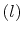positions (Figure 5.1) couple to the cavity. Excitations are introduced into the cavity model (4.13) by vertical currents on ports between the upper and lower plane.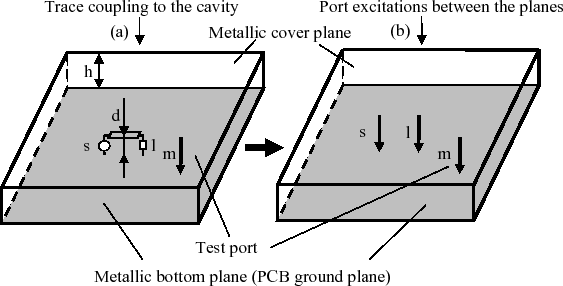The port excitation currents in Figure 5.1(b) are the trace currents at the source (s) and the load (l) in Figure 5.1 (a) multiplied by the constant factor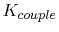. This mode conversion factor accounts for the coupling from the trace to the common mode cavity field. Port m in Figure 5.1 has been introduced for the voltage measurement between the planes. With (4.17), the voltage on the test port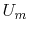can be expressed by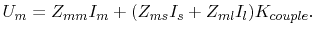(5.1)

Indices are assigned to the port definition in Figure 5.1. The trace voltages and currents at the port positions s and l in Figure 5.1 (a) can be expressed by transmission line theory with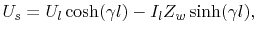(5.2)

and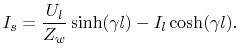(5.3)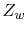is the characteristic impedance of the trace,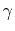is the complex propagation factor of the trace, and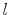is the trace length. The negative sign of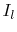in (5.2) and (5.3) is consistent with the definition of the same current flow direction for all ports. The relation of the load voltage to the load current is given by the load impedance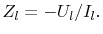(5.4)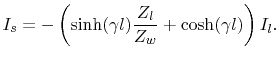(5.5)

Since port m acts like a voltage probe, the current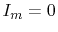. With,  (5.1), and (5.5) the transfer impedance of the current at the source of the trace to the voltage on the test port becomes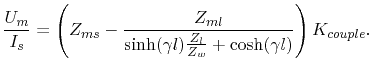(5.6)

Equation (5.6) describes the voltage between the two planes at the test port m for a given trace source current. The vertical connections of the trace have to be considered by using an effective trace length(Figure 5.2) in (5.6).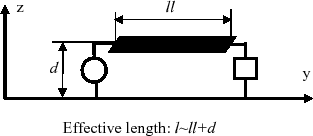Since (5.6) does not consider the back coupling from the cavity field to the trace, it is valid in case of emission simulation, where the trace currents are determined by the trace geometry above the ground plane, the source, and the load. The dielectric layers in printed circuit boards are usually thin, compared to the distance from the ground plane to a parallel metallic enclosure cover. Therefore, the influence of the metallic cover plane on the characteristic impedance of the traces is negligible and the currents on the traces can be simulated with this characteristic impedance, the driver, and the load models. Characteristic impedances with and without the metallic cover plane can be compared with numerical simulation.
Although the back coupling is small, it has to be considered for susceptibility simulations of sensitive devices. In this case, the transmission line equations of the trace have to be integrated into the impedance matrix (4.17) with additional back coupling terms. However, this work concentrates on the emission simulation, where the back coupling can be neglected.
Equation (5.6) is an example of the introduction of one trace into a cavity model with one measurement port. Multiple traces can be considered in a cavity model by the introduction of trace currents from (5.5) to (4.17) as excitations.
The coupling factorin (5.6) is obtained by mode decomposition, or expressed analytically as distance ratio. Both methods are described in the following sections.

Subsections

C. Poschalko: The Simulation of Emission from Printed Circuit Boards under a Metallic Cover